Next: Exercises Up: Examples Previous: Sinusoidal waveshaping: evenness and   Contents   Index

## Phase modulation and FM

Example E08.phase.mod.pd, shown in Figure 5.15, shows how to use Pd to realize true frequency modulation (part a) and phase modulation (part b). These correspond to the block diagrams of Figure 5.8. To accomplish phase modulation, the carrier oscillator is split into its phase and cosine lookup components. The signal is of the form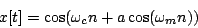whereis the carrier frequency,is the modulation frequency, and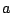is the index of modulation--all in angular units.We can predict the spectrum by expanding the outer cosine:Plugging in the expansions from Pageand simplifying yields:So the components are centered about the carrier frequencywith sidebands extending in either direction, each spacedfrom the next. The amplitudes are functions of the index of modulation, and don't depend on the frequencies. Figure 5.16 shows some two-operator phase modulation spectra, measured using Example E09.FM.spectrum.pd.Phase modulation can thus be seen simply as a form of ring modulated waveshaping. So we can use the strategies described in Section 5.2 to generate particular combinations of frequencies. For example, if the carrier frequency is half the modulation frequency, you get a sound with odd harmonics exactly as in the octave dividing example (Figure 5.10).

Frequency modulation need not be restricted to purely sinusoidal carrier or modulation oscillators. One well-trodden path is to effect phase modulation on the phase modulation spectrum itself. There are then two indices of modulation (call themand) and two frequencies of modulation (and) and the waveform is:To analyze the result, just rewrite the original FM series above, replacing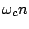everywhere with. The third positive sideband becomes for instance: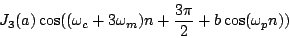This is itself just another FM spectrum, with its own sidebands of frequency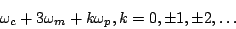having amplitudeand phase[Leb77]. Example E10.complex.FM.pd (not shown here) illustrates this by graphing spectra from a two-modulator FM instrument.

Since early times [Sch77] researchers have sought combinations of phases, frequencies, and modulation indices, for simple and compact phase modulation instruments, that manage to imitate familiar instrumental sounds. This became a major industry with the introduction of commercial FM synthesizers.Next: Exercises Up: Examples Previous: Sinusoidal waveshaping: evenness and   Contents   Index
Miller Puckette 2006-12-30